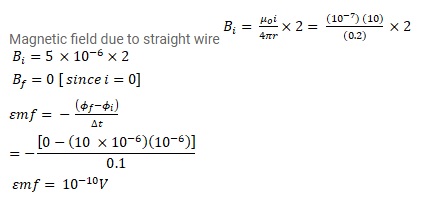# A conducting circular loop of area

Question:

A conducting circular loop of area $1 \mathrm{~mm}^{2}$ is placed coplanarly with a long, straight wire at a distance of $20 \mathrm{~cm}$ from it. The straight wire carries an electric current which changes from $10 \mathrm{~A}$ to zero in $0.1 \mathrm{~s}$. Find the average emf induced in the loop in $0.1 \mathrm{~s}$

Solution: